GRE Subject Test: Math : Graphing Functions

Example Questions

Example Question #1 : Graphing Functions

At what point does the line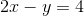cross the y-axis?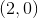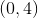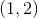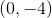Explanation:

Step 1: Rearrange the terms into the form y=mx+b. Move theto the other side.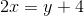Step 2: Move the 4 to the other side.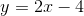Step 3: When the line crosses the y-axis, the x value is zero. We will plug infor x and find the y value.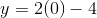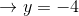So, the point where this line crosses the y-axis isAll GRE Subject Test: Math Resources